# Transformation T:R' -»R',T(x,y, z) = (x+y,x-z)nd v=< 1,-1,2 > 8. ar iven B <1,1,1>,< 1,0,1 >&...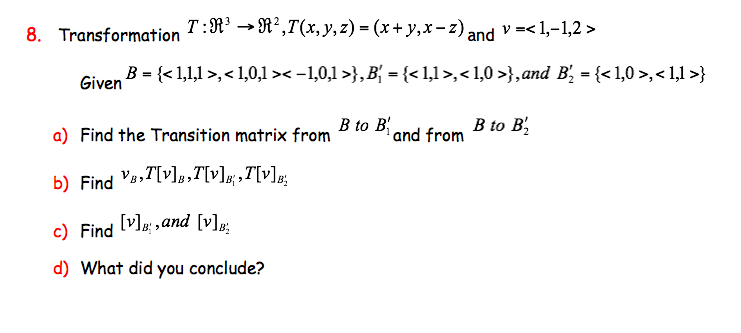Transformation T:R' -»R',T(x,y, z) = (x+y,x-z)nd v= 8. ar iven B ,},B^ = {,},and B, = {,} B to Biand from B to B2 a) Find the Transition matrix from b) Find v],T[v];,7[v] c) Find v,and [v]p d) What did you conclude?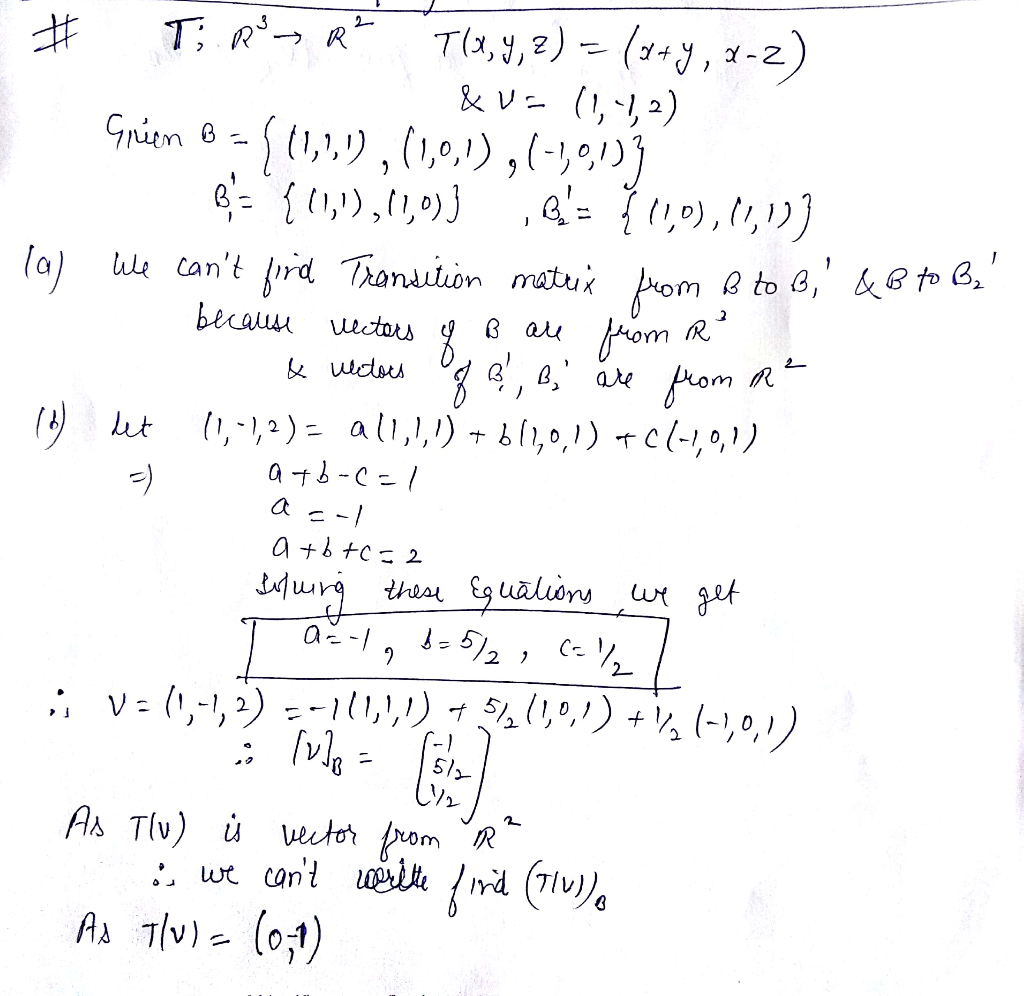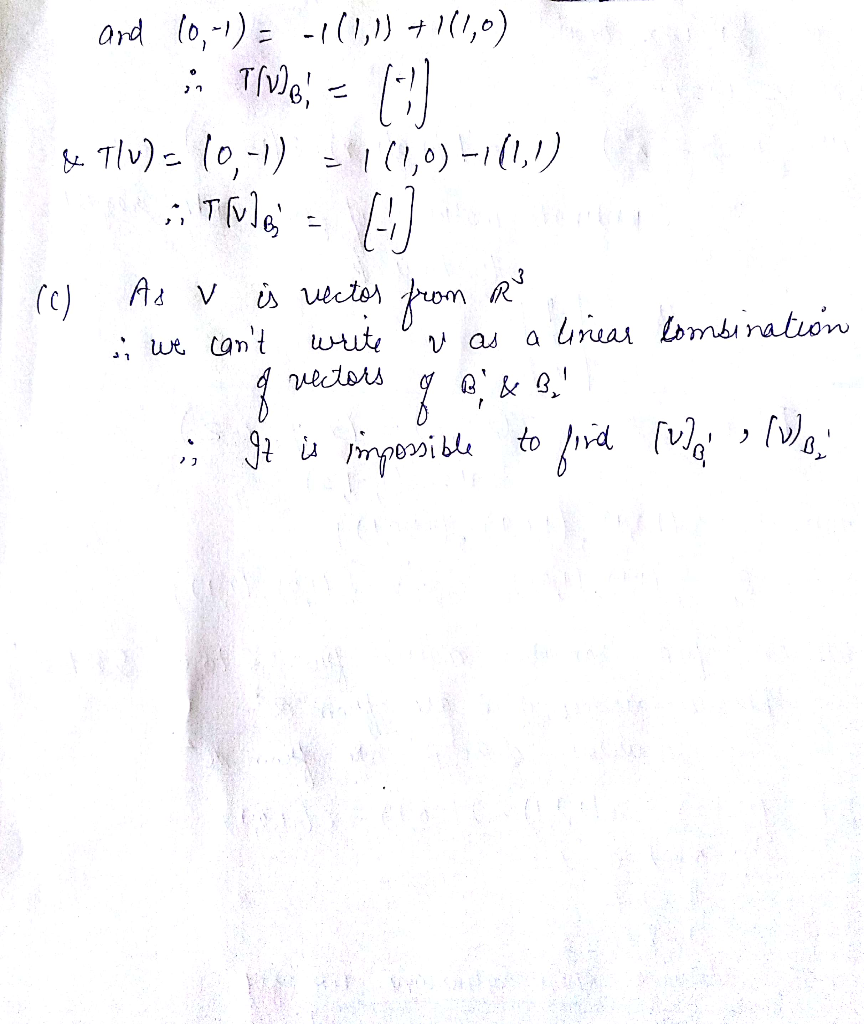##### Add Answer of: Transformation T:R' -»R',T(x,y, z) = (x+y,x-z)nd v=< 1,-1,2 > 8. ar iven B <1,1,1>,< 1,0,1 >&...
Similar Homework Help Questions
• ### Linear algebra, I need someone to tell me how to get T(1)=1,1,1 T(x)=-1,0,1 T(x^2)=1,0,1 T(x^3)=-1,0, 1...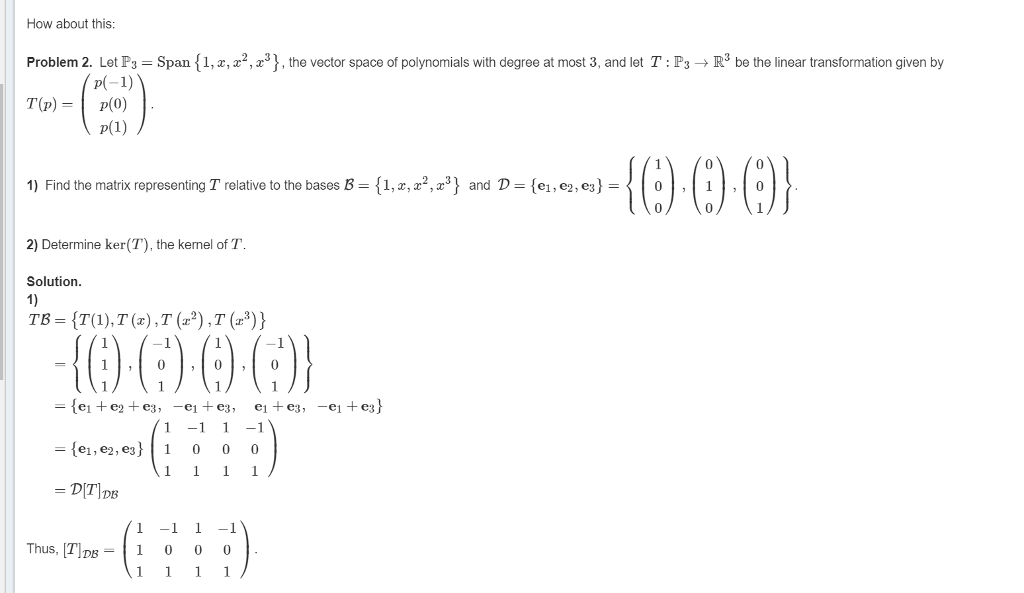Linear algebra, I need someone to tell me how to get T(1)=1,1,1 T(x)=-1,0,1 T(x^2)=1,0,1 T(x^3)=-1,0, 1 I don't have any clue to find this. please follwo the comment WHAT FORMULA SHOULD I PLUG IN WHEN I PLUG IN T(1), T(X)...... How about this: Problem 2. Let P3 = Span {1,2,22,23 , the vector space of polynomials with degree at most 3, and let T : P3 → R3 be the linear transformation given by T(p)p(0) 1000 1) Find the matrix...

• ### T:R^3 -> R^2 is given by T(x,y,z) = (x+2y,y-z). Find the matrix of T relative to the standard...

T:R^3 -> R^2 is given by T(x,y,z) = (x+2y,y-z).Find the matrix of T relative to the standard basis.Use that matrix to find the kernel of T.please show step by step solution

• ### 12: Find a basis B for R', such that the matrix for the linear transformation T: R' R', T(x,y,z)-(2x-2z,2y-2z,3x-3z) relative to B is diagonal. 12: Find a basis B for R', such th...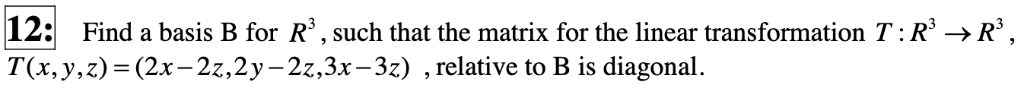12: Find a basis B for R', such that the matrix for the linear transformation T: R' R', T(x,y,z)-(2x-2z,2y-2z,3x-3z) relative to B is diagonal. 12: Find a basis B for R', such that the matrix for the linear transformation T: R' R', T(x,y,z)-(2x-2z,2y-2z,3x-3z) relative to B is diagonal.

• ### Consider the transformation x = v cos :2πu, y =v sin 2πι. (a) Describe the image...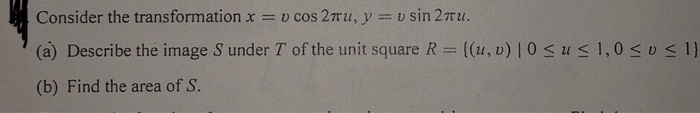Consider the transformation x = v cos :2πu, y =v sin 2πι. (a) Describe the image S under T of the unit square R = {(u, v) | 0 1,0 << 1 u (b) Find the area of S. Consider the transformation x = v cos :2πu, y =v sin 2πι. (a) Describe the image S under T of the unit square R = {(u, v) | 0 1,0

• ### If A is an m × n matrix, and x is an n × 1 vector, then the linear transformation y = Ar maps R" to R", so the...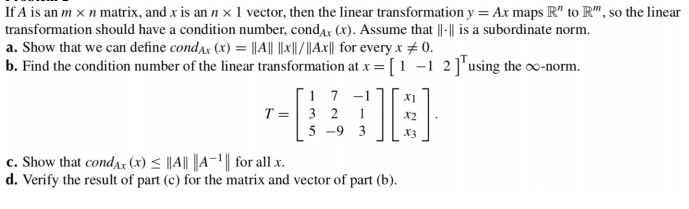If A is an m × n matrix, and x is an n × 1 vector, then the linear transformation y = Ar maps R" to R", so the linear transformation should have a condition number, condar (x). Assume that |I-ll is a subordinate norm. a. Show that we can define condar (x)-|All llrI/IAxll for every x 0. b. Find the condition number of the linear transformation at[ 2] using the oo-norm. c. Show that condAr(x) IIA for all x....

• ### If A is an m × n matrix, and x is an n × 1 vector, then the linear transformation y = Ar maps R" to R", so the...If A is an m × n matrix, and x is an n × 1 vector, then the linear transformation y = Ar maps R" to R", so the linear transformation should have a condition number, condar (x). Assume that |I-ll is a subordinate norm. a. Show that we can define condar (x)-|All llrI/IAxll for every x 0. b. Find the condition number of the linear transformation at[ 2] using the oo-norm. c. Show that condAr(x) IIA for all x....

• ### 5. Consider a vector space V = {00 + 0 + ar? + 13. 1 40,,...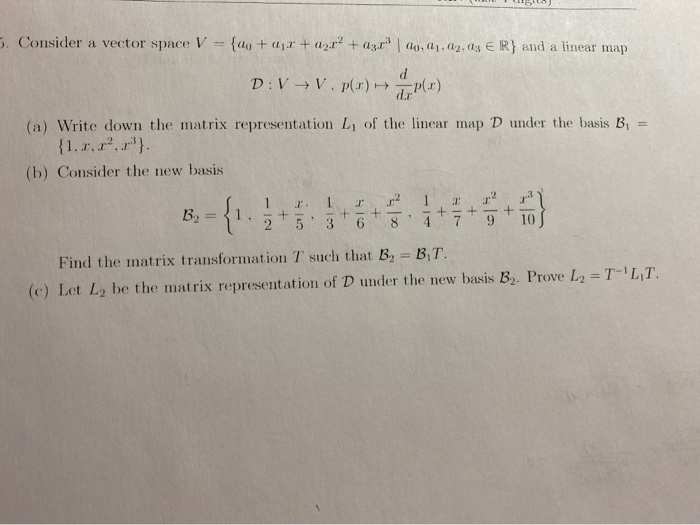5. Consider a vector space V = {00 + 0 + ar? + 13. 1 40,, 02, 03 € R} and a linear map D:V+V. P(1) - P(1) (a) Write down the matrix representation L of the linear map D under the basis B, = (b) Consider the new basis B2 = 31.5 +5.368.479 10 Find the matrix transformation T such that B, BT. (c) Let L, be the matrix representation of D under the new basis B. Prove L2...

• ### Linear Algebra! Practice exam #1 question 1 Thanks for sloving! 1- Transformations (3 points each) a) Given a linear transformation T :N" N" T(x,y)-(x-y,x+y) and B= {< l, 0>.< 1,...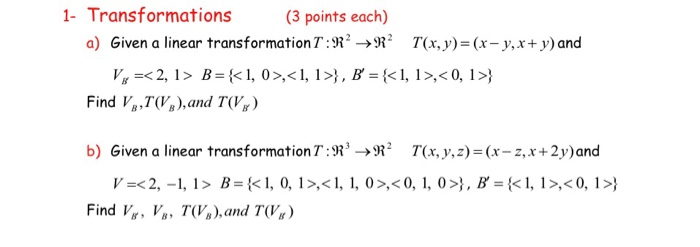Linear Algebra! Practice exam #1 question 1 Thanks for sloving! 1- Transformations (3 points each) a) Given a linear transformation T :N" N" T(x,y)-(x-y,x+y) and B= {< l, 0>.< 1,1 >} , B = {< l, l>,< 0, l>} V,-< 2, l> Find V,T,and TVg) b) Given a linear transformation T:n'->n2 T(x,y,2)-(x-z,x +2y)and V =< 2,-I, I> B= {<l, 0, 1>.< 1, 1, 0 >, < 0, l, 0 >}, B' = {<l, l >, < 0, 1 >} Find...

• ### For the operator T e L(R2, R2) defined by T(x,y,z) = (x + y, -2x + 4y)

(a) For the operator T ε L(R2, R2) defined by T(x,y,z) = (x + y, -2x + 4y), determine [T]B, whereB = {(1,1)T, (1,2)T}.(b) Now, let T be the operator on R3 defined by T(x,y,z) = (x - y, y - x, x - z) and considerv = (1,1,2)T and B = {(1,0,1)T, (0,1,1)T, (1,1,0)T}.1. Determine [T]B and [v]B2. Compute [T(v)]B, and then verify that [T]B[v]B = [T(v)]B.

• ### tks for the help (thumb) 7. (a) . Illustrate diagram the image of the unit square on a 1,0 y 1 {(r,y) 0 when transforme...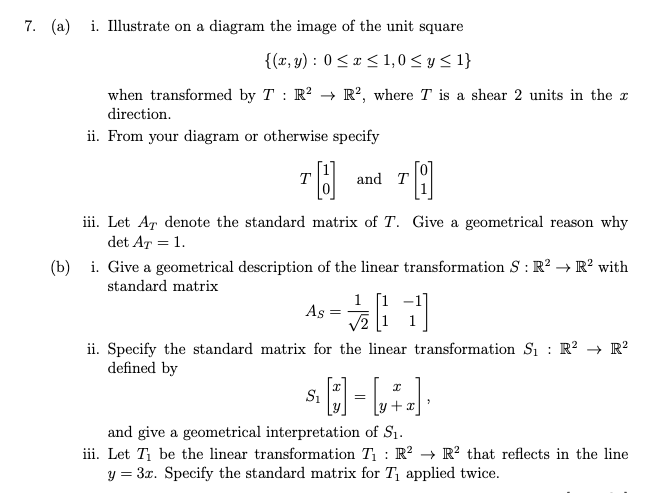tks for the help (thumb) 7. (a) . Illustrate diagram the image of the unit square on a 1,0 y 1 {(r,y) 0 when transformed by T : R2 direction ii. From your diagram or otherwise specify R2, where T is a shear 2 units in the r 0 and T T ii. Let Ar denote the standard matrix of T. Give det Ar 1 geometrical why reason a . Give a geometrical description of the linear transformation S: R2...

Need Online Homework Help?